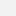# Humboldt-Universität zu Berlin

„The KPZ Equation on the Real Line“

## „The KPZ Equation on the Real Line“

Tommaso Cornelis Rosati promoviert in Mathematik an der Humboldt-Universität. Für seine Masterarbeit wurde er mit dem Humboldt-Preis 2018 ausgezeichnet.Tommaso Cornelis Rosati
Foto: privat

### Zusammenfassung

In 1986 the physicists Kardar, Parisi, and Zhang introduced a stochastic partial differential equation (SPDE) for the fluctuations of the boundary height h separating two competing materials. This model found a wide range of applications, from describing the expansion of a forest fire to the interface separating water from ice. The equation reads:

(t-x2)h=(xh)2+ξ,    h(0)=h0

Where ξ is white noise, namely a random external forcing, which acts independently at any given point in time and space.

The large interest behind this equation has driven mathematical research in the field of SPDEs. Indeed the chaotic behavior of the noise makes the model complicated, as randomness is not only a source of uncertainty, but also of irregularity and roughness.

In 2011, Martin Hairer achieved a breakthrough in the solution theory for the KPZ, a first step towards a celebrated theory called of regularity structures. A similar approach was developed at the same time and independently in the works by Gubinelli, Imkeller and Perkowski. In a nutshell, these theories rely on a fine separation between the uncertainty and the irregularity of the noise and their success lies in the ability to treat the latter independently of the first one.

In this work, we address the open problem of solving the KPZ equation on the real line, i.e. on R+×R. Until now, existence of solutions was proven only on bounded regions in space. This is not completely satisfactory, since mainly the large scales behavior of the solution is interesting from the physical viewpoint.

The reason why the problem was still open is rather technical: the noise becomes increasingly intense on large scales, breaking down the mathematics in the existence proofs.

The approach in this thesis is to show that solutions to the KPZ on a bounded region converge to a solution on the whole space as we enlarge the region. This relies on a priori estimates, which guarantee that no explosion occurs. To find such estimates we use a remarkable formulation of the solution to the KPZ via an optimal control problem. This representation is a consequence of a fundamental link between the study of stochastic processes and the theory of partial differential equations. This link permeates the whole work, as we are able to apply the results in and regarding the solution to certain classes of stochastic differential equations. Contrary to how we started, we now add random structures at a point where everything is deterministic.

Eventually, we can prove existence of a solution to the KPZ equation as well as construct of the random directed polymer measure, which is interesting from a physical point of view and allows us to recover the link to the optimal control problem.